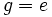# Nonstandard definitions of normal subgroup

## Definition in terms of measures of deviation from normality

1. A normal subgroup is a subgroup whose normalizer is the whole group.
2. A normal subgroup is a subgroup that equals its normal core.
3. A normal subgroup is a subgroup that equals its normal closure.
4. A normal subgroup is a subgroup that equals each of its conjugate subgroups.

## Definition in terms of group actions

1. A normal subgroup is a fixed point under the action of the group on the set of its subgroups by conjugation.

## Definitions from category theory

1. A normal subgroup is a normal monomorphism (i.e., it is a monomorphism that occurs as the kernel of some epimorphism). Here, we are thinking of subgroups not as subsets but rather as inclusion maps.

## Definitions from universal algebra

1. A normal subgroup is a kernel in the variety with zero of groups.
2. A normal subgroup is an ideal in the variety with zero of groups.

## Definition in terms of linear representations

1. A subgroup$N$ of a group$G$ is termed normal in$G$ if and only if there exists a linear representation of$G$ over a field of characteristic zero, with the property that the character of the representation is nonzero on all elements of$N$, and zero on all elements outside$N$.
2. A subgroup$N$ of a group$G$ is termed normal in$G$ if and only if the trivial linear representation of$N$ over characteristic zero, induces a representation of$G$ (by induction of representations) that is zero on all elements outside$N$.

## Definitions in terms of generating sets

1. A subgroup$N$ of a group$G$ is normal in$G$ if, whenever$A$ is a generating set of$G$ and$B$ is a generating set of$N$,$aba^{-1} \in N$ and$a^{-1}ba \in N$ for all$a \in A, b \in B$. (Note that this is the definition used to test normality -- Further information: Normality testing problem
2. Suppose$G$ is a group and$N$ is a subgroup. Suppose we quotient out$G$ by relations of the form$g = e$ for all$g \in N$. Then$N$ is normal if and only if the only elements that become trivial in the quotient, are those that originally came from$N$.

## Definition via function restriction expression

### Extensible automorphism

Further information: Extensible automorphism, extensible implies inner, quotient-pullbackable implies inner

An extensible automorphism of a group is an automorphism that can be extended to an automorphism of any bigger group containing it.

It turns out that an automorphism of a group is extensible iff it is inner. Thus, normality can be expressed as the invariance property with respect to extensible automorphisms:

Extensible automorphism$\to$ Function

In other words, a subgroup$H$ of a group$G$ is normal in$G$ if and only if every extensible automorphism of$G$ sends$H$ to itself.

### Normal automorphisms, monomial automorphisms, strong monomial automorphisms, and weakly normal automorphisms

• Normal automorphism$\to$ Function
• Normal automorphism$\to$ Endomorphism
• Normal automorphism$\to$ Automorphism
• Weakly normal automorphism$\to$ Function
• Weakly normal automorphism$\to$ Endomorphism
• Weakly normal automorphism$\to$ Injective endomorphism
• Monomial automorphism$\to$ Function
• Monomial automorphism$\to$ Endomorphism
• Monomial automorphism$\to$ Injective endomorphism
• Strong monomial automorphism$\to$ Function
• Strong monomial automorphism$\to$ Endomorphism
• Strong monomial automorphism$\to$ Automorphism

## Circular definitions

1. A normal subgroup is a subgroup such that every characteristic subgroup of it is normal in the whole group. Further information: characteristic of normal implies normal
2. A normal subgroup is a subgroup that is normal in every intermediate subgroup. Further information: Normality satisfies intermediate subgroup condition
3. A normal subgroup is a subgroup whose intersection with every normal subgroup is normal. Further information: Normality is strongly intersection-closed
4. A subgroup$H$ is normal in$G$ if there exists a group$K$ containing$G$, such that$H$ is normal in$G$. Further information: Normality satisfies intermediate subgroup condition